## Dynamic Time Warping with Financial Time SeriesIn one of the ML books I was going through from Packt Publishing (I can’t figure out which book it was–it has been a while), I saw a cool example of using dynamic time warping (DTW) for detecting the similarities of music tracks. Audio data is a type of time series. Comparing the distances between two time series at each point in time can provide a sense of whether the two music tracks (time series) are more or less similar depending on the distance.

I wondered what would happen if I applied DTW to some basic financial time series and came up with some interesting visualization results.

The Jupyter notebook and full source code can be accessed on GitHub:

https://github.com/groxli/notebooks/blob/master/dtw_with_financial_time_series.ipynb

#### Package Dependencies

We’ll use a couple of Python packages that you may need to install: pandas_datareader and dtw.

Assuming you have the Python package installer configured for your environment, you can easily install the extra packages:

pip install pandas_datareader
pip install dtw

#### Import the packages

In a .py file or a Jupyter notebook, import the following:### Data Getter

This function loops through a list of ticker symbols and retrieves the time series data from Yahoo! Finance.

#### Inputs

The following function takes 3 inputs:

• A Python list of ticker symbols.
• Start Date in Python datetime format.
• End Date in Python datetime format.

#### Outputs

The function returns a Python dictionary containing time series data for each symbol. The keys to the dictionary are the symbols.#### List of Ticker Symbols

Here is a list of some random symbols from different industries. Depending on your connection speed, it could take a while to download one year’s data for a given symbol. On my home network one symbol’s series only takes a couple of seconds while on a shared network the download can take as much as 10 seconds per symbol.For starters, let’s pick a couple of tickers from a similar industry: MSFT (Microsoft) and AAPL (Apple).

After we set the x and y symbols, convert the Pandas data frames into Numpy arrays. We will use the Numpy arrays for doing the number crunching and plotting.### Call the DTW Function

Here is where the number crunching occurs.

###### Inputs

The dtw( ) function takes the two time series (x and y in this case) along with a function for calculating the distance between the two time series. In this case, the distance function is simply Numpy’s norm( ) function, but it is possible to use more sophisticated distance functions. (I’m not sure what’s out there in terms of alternatives, but I mention it because you will probably get significantly different outputs depending on the distance function.)

###### Python’s lambda

The first two input parameters are straightforward. Let’s look at the distance function (which is the third input parameter.) The ‘dist=’ is the input label and assignment operator. lambda is Python’s nice way of declaring that one or more variables or objects (such as a Python list) will need to be parsed by some other sort of function, in this case Numpy’s norm( ) function. lambda functions can be a bit confusing depending on other language backgrounds, but if you think of Python’s lambda as a nice way to avoid writing unnecessary for-loops, you should be in good shape.###### Outputs

The dtw( ) function (at least, the particular implementation we are using) returns the following:

• minimum distance
• the cost matrix
• the accumulated cost matrix
• wrap path.

We are only interested in the accumulated cost matrix which is derived from the distance function we passed into dtw( ). The minimum distance, which is a scalar rather than a matrix, could be interesting when comparing many time series.

### Visualization

We’ll use the matplotlib package. Seaborn is another visualization package that rides on top of matplotlib and provides a rich set of convenience functions for enriched visualizations.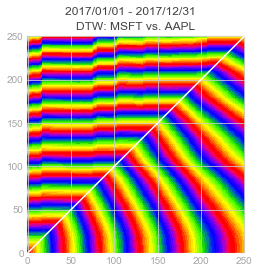The 250 x 250 grid is the number of steps in the time series. In this case, we took 1 year of market data, hence the 250 days.

### Sanity Check

Looking at the graph, it becomes clear there is some sort of divergence that happens over time between the two time series. Let’s look at a regular line plot. (Since our time series are in a pandas DataFrame, it is super-easy!)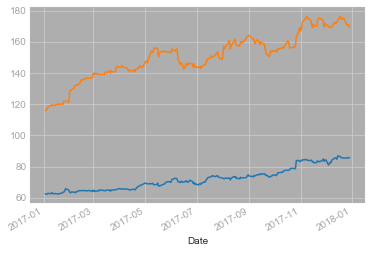### Comparing Time Series from Different Industry Sectors

Let’s compare closing prices of McDonalds and Apple. There should be some more interesting visualization results assuming there is not a tight correlation between the two series.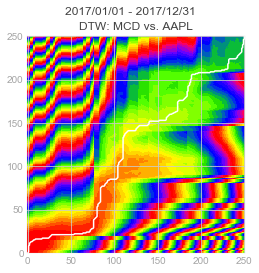Wow. This is interesting. There is a lot more divergence than the MSFT vs. AAPL time series. Let’s do another sanity check with a line plot: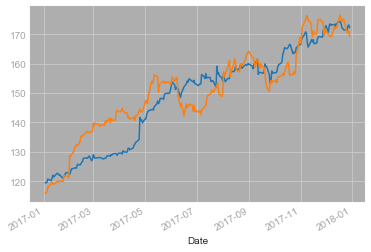The line plot shows that while one time series is experiencing market support, the other experiences market resistance, and over time there is crossover. This explains the wild, yet oddly rhythmic, visual patterns in the DTW plot.

### Time Shifting

It is also interesting to see what happens when a single time series is compared against itself. This first plot is for Deutsche Bank’s closing prices, no time shifting: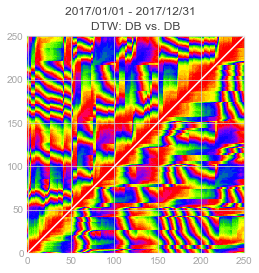Although the visuals are a bit psychedelic, there is an obvious symmetry shared between the x and y series.

#### Applying the Time Shift

Let’s shift the time series and see what happens. Numpy’s array slicing make it easy to do.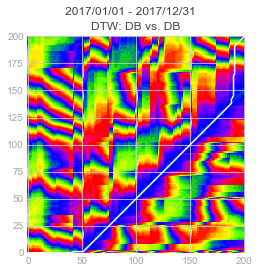Perturbing a symmetrical set of time series results might also provide interesting insights depending on the use case.